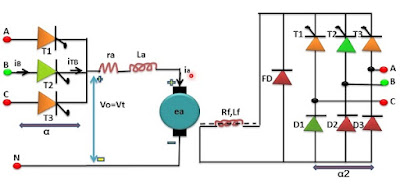## Search This Blog

### Three phase half wave converter drives

• Three phases half wave converter drive consisting of two converts and a separately excited dc motor.
• The armature circuit of the motor is fed through a three-phase half wave converter whereas its field is energized through a three-phase semi-converter.
• Two quadrant operation can also be obtained from three phases half wave converter drive in case motor field winding is energized from a single phase or three phases full converter.Three phases half wave converter drive  V0 = Vt =  3Vml / 2∏ * Cos ɖ  for the  0< ɖ <  ∏ The voltage expression is valid only for the contentious armature current. for three phase semi-converter, the average value field voltage from the equation given by this equation given below,                                         Vf =  3Vml/2∏ * (1+Cos  ɖ1 ) for 0<  ɖ1 <  ∏   Vml = Is the maximum value of line voltage and ɖ is the firing angle for converter 1.
Application :

A three-phase half wave converter drive is not normally used in the industrial application as introduce dc component in the ac supply lines.
• Three phases half wave converter drive consisting of two converts and a separately excited dc motor.
• The armature circuit of the motor is fed through a three-phase half wave converter whereas its field is energized through a three-phase semi-converter.
• Two quadrant operation can also be obtained from three phases half wave converter drive in case motor field winding is energized from a single phase or three phases full converter.Three phases half wave converter drive  V0 = Vt =  3Vml / 2∏ * Cos ɖ  for the  0< ɖ <  ∏ The voltage expression is valid only for the contentious armature current. for three phase semi-converter, the average value field voltage from the equation given by this equation given below,                                         Vf =  3Vml/2∏ * (1+Cos  ɖ1 ) for 0<  ɖ1 <  ∏   Vml = Is the maximum value of line voltage and ɖ is the firing angle for converter 1.
Application :

A three-phase half wave converter drive is not normally used in the industrial application as introduce dc component in the ac supply lines.